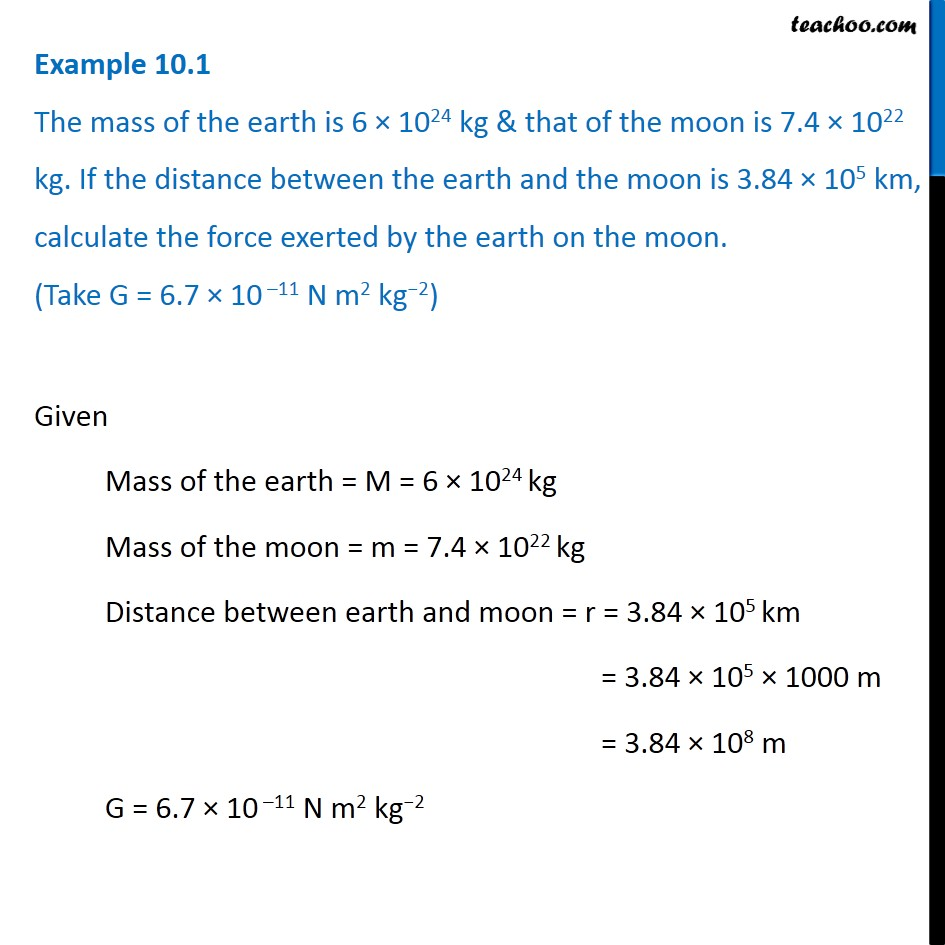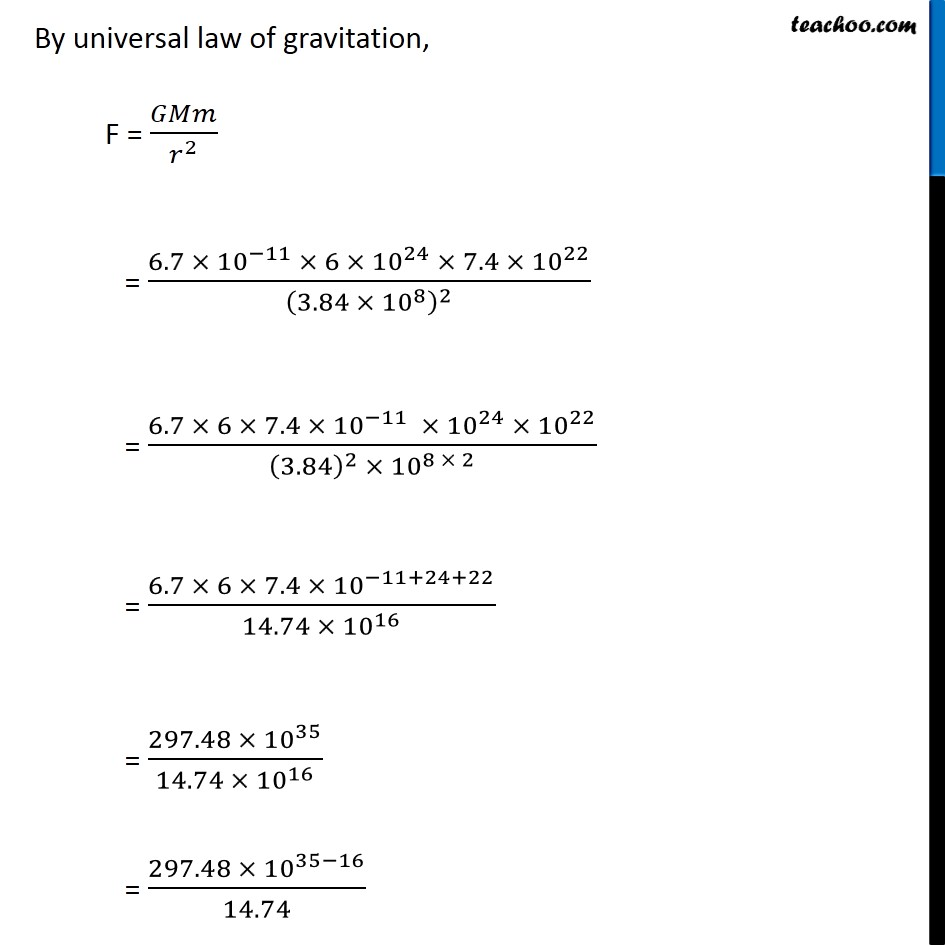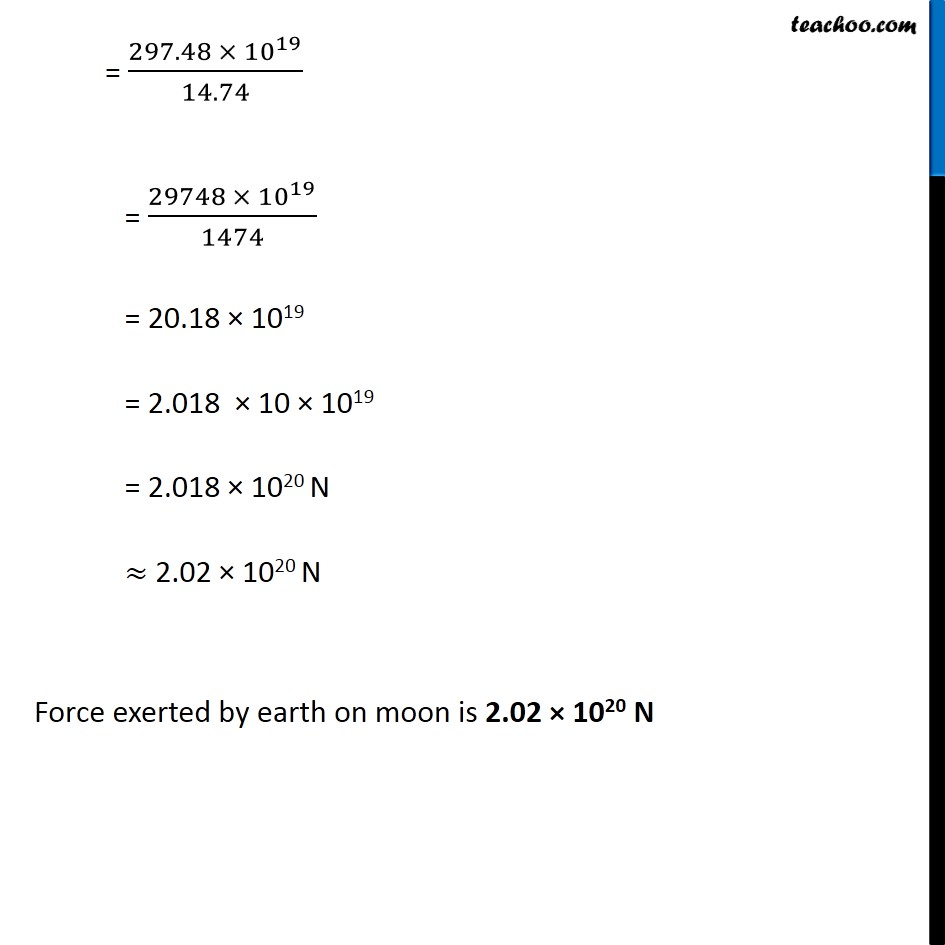Examples from NCERT Book

Class 9
Chapter 10 Class 9 - GravitationLearn in your speed, with individual attention - Teachoo Maths 1-on-1 Class

### Transcript

Example 10.1 The mass of the earth is 6 × 1024 kg & that of the moon is 7.4 × 1022 kg. If the distance between the earth and the moon is 3.84 × 105 km, calculate the force exerted by the earth on the moon. (Take G = 6.7 × 10 –11 N m2 kg−2) Given Mass of the earth = M = 6 × 1024 kg Mass of the moon = m = 7.4 × 1022 kg Distance between earth and moon = r = 3.84 × 105 km = 3.84 × 105 × 1000 m = 3.84 × 108 m G = 6.7 × 10 –11 N m2 kg−2 By universal law of gravitation, F = 𝐺𝑀𝑚/𝑟^2 = (6.7 × 〖10〗^(−11) × 6 × 〖10〗^24 × 7.4 × 〖10〗^22)/(3.84 ×〖 10〗^8 )^2 = (6.7 × 6 × 7.4 × 〖10〗^(−11) × 〖10〗^24 × 〖10〗^22)/((3.84)^2 × 〖10〗^(8 × 2) ) = (6.7 × 6 × 7.4 × 〖10〗^(−11+24+22))/(14.74 × 〖10〗^16 ) = (297.48 × 〖10〗^35)/(14.74 × 〖10〗^16 ) = (297.48 × 〖10〗^(35−16))/14.74 = (297.48 × 〖10〗^19)/14.74 = (29748 × 〖10〗^19)/1474 = 20.18 × 1019 = 2.018 × 10 × 1019 = 2.018 × 1020 N ≈ 2.02 × 1020 N Force exerted by earth on moon is 2.02 × 1020 N